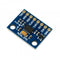# Overview

The final key to the puzzle lies within the ADXL345 accelerometer. The easiest way to obtain a pedometer is buying one. But where’s the challenge in that?

For a more detailed ADXL345 Accelerometer visit my good friend, Eddyson’s, post here :

# Application Description

There are different types of pedometer available in the market. Some are dedicated wearables, and some are phone applications that uses the phone’s sensors. Pedometer apps for Android for example are abundant as listed here: https://joyofandroid.com/best-pedometer-apps-for-android

Nonetheless, either wearables or phone apps, they serve the same purpose : To measure a person’s step.

The magic to make the accelerometer work as a pedometer lies within the code. It uses an adaptive peak detection so that each back-and-forth movement can be recognized as a step.

Before you upload the code here, be sure to calibrate your Accelerometer ! Otherwise it will be highly inaccurate in recognizing your steps !

## Calibration

1. The Pedometer Calibration is very simple. Upload the code to your Arduino, and Note the threshold value.
2. Walk around a few steps, or move the accelerometer a few times. Increase or decrease the threshold value, where a movement should be recognized as a step.
3. You are done !

# Set-up the Hardware

Similiar to Eddyson’s set-up the hardware !# Code

The code is very similar to the linked post, except that function ArduinoPedometer() is added.

```#include <Wire.h>

#define TO_READ (6)        //num of bytes we are going to read each time (two bytes for each axis)

#define offsetX   -10.5       // OFFSET values
#define offsetY   -2.5
#define offsetZ   -4.5

#define gainX     257.5        // GAIN factors
#define gainY     254.5
#define gainZ     248.5

byte buff[TO_READ] ;    //6 bytes buffer for saving data read from the device
char str;                      //string buffer to transform data before sending it to the serial port

int x,y,z;

int xavg, yavg,zavg, steps=0, flag=0;
int xval={0}, yval={0}, zval={0};
int threshhold = 60.0;

void setup()
{
Wire.begin();        // join i2c bus (address optional for master)
Serial.begin(9600);  // start serial for output

writeTo(DEVICE, 0x2D, 0);
writeTo(DEVICE, 0x2D, 16);
writeTo(DEVICE, 0x2D, 8);
}

void loop()
{

//each axis reading comes in 10 bit resolution, ie 2 bytes.  Least Significat Byte first!!
//thus we are converting both bytes in to one int
x = (((int)buff) << 8) | buff;
y = (((int)buff)<< 8) | buff;
z = (((int)buff) << 8) | buff;

//we send the x y z values as a string to the serial port
//  sprintf(str, "%d %d %d", x, y, z);
//  Serial.print(str);
//  Serial.print(10, byte());
x = ArduinoPedometer();
Serial.println(x);
//It appears that delay is needed in order not to clog the port
delay(100);
}

//---------------- Functions
//Writes val to address register on device
void writeTo(int device, byte address, byte val) {
Wire.beginTransmission(device); //start transmission to device
Wire.write(val);        // send value to write
Wire.endTransmission(); //end transmission
}

//reads num bytes starting from address register on device in to buff array
Wire.beginTransmission(device); //start transmission to device
Wire.endTransmission(); //end transmission

Wire.beginTransmission(device); //start transmission to device
Wire.requestFrom(device, num);    // request 6 bytes from device

int i = 0;
while(Wire.available())    //device may send less than requested (abnormal)
{
i++;
}
Wire.endTransmission(); //end transmission
}

//Get pedometer.

int ArduinoPedometer(){
int acc=0;
int totvect={0};
int totave={0};
int xaccl={0};
int yaccl={0};
int zaccl={0};
for (int i=0;i<15;i++)
{
xaccl[i]= x;
delay(1);
yaccl[i]= y;
delay(1);
zaccl[i]= z;
delay(1);
totvect[i] = sqrt(((xaccl[i]-xavg)* (xaccl[i]-xavg))+ ((yaccl[i] - yavg)*(yaccl[i] - yavg)) + ((zval[i] - zavg)*(zval[i] - zavg)));
totave[i] = (totvect[i] + totvect[i-1]) / 2 ;
delay(150);

//cal steps
if (totave[i]>threshhold && flag==0)
{
steps=steps+1;
flag=1;
}
else if (totave[i] > threshhold && flag==1)
{
//do nothing
}
if (totave[i] <threshhold  && flag==1)
{
flag=0;
}
// Serial.print("steps=");
// Serial.println(steps);
return(steps);
}
delay(100);
}```

# Code Breakdown

`int threshhold = 60.0;`

We are interested in this variable, since this determines when to register a movement as a step.

```x = (((int)buff) << 8) | buff;
y = (((int)buff)<< 8) | buff;
z = (((int)buff) << 8) | buff;```

Since we are receiving a chunk of data, we will separate/parse the data into different variables.

`totvect[i] = sqrt(((xaccl[i]-xavg)* (xaccl[i]-xavg))+ ((yaccl[i] - yavg)*(yaccl[i] - yavg)) + ((zval[i] - zavg)*(zval[i] - zavg)));`

This formula is the length of a line segment. We will be using this to detect a movement/step.

Here’s a discussion on different pedometer algorithms : https://stackoverflow.com/questions/20324356/how-to-calculate-exact-foot-step-count-using-accelerometer-in-android.

We’ll use the (1) as our algorithm.

# Conclusion

The ADXL345 accelerometer is a versatile device that can be used in many applications. If a time element is used, it can be used to determine the distance traveled.